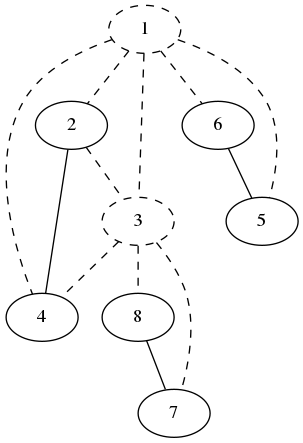시간 제한메모리 제한제출정답맞힌 사람정답 비율
3 초 1024 MB82240.000%

## 문제

Vasya likes trees and hates cycles in graphs very much. Every time when he faces a cactus problem at a contest, he gets upset, and then he starts a discussion at one forum which is well-known in the community.

Today, during the night before an important contest at the training camp, Vasya has a nightmare. In this nightmare he sees a connected undirected graph with a lot of cycles: cycles with cycles inside with cycles inside... The graph is so terrible that for every simple cycle with at least four vertices, there is an edge which connects two non-adjacent vertices of the cycle.

The only thing that Vasya can do is to delete a vertex and all the edges that are incident to this vertex. Help Vasya to delete some vertices, so that the number of remaining vertices is as large as possible, but there are no cycles at all in the remaining graph. Note that the graph may become disconnected after deletion of vertices.

## 입력

On the first line, you are given two integers $n$ and $m$: the numbers of vertices and edges in the graph ($1 \le n, m \le 200\,000$).

Each of the next $m$ lines contains a pair of integers $u$ and $v$: the numbers of vertices that are connected by an edge ($1 \le u, v \le n$).

It is guaranteed that the graph is connected, there are no loops, no multiple edges, and for every simple cycle with at least four vertices, there is an edge which connects two non-adjacent vertices of the cycle.

## 출력

On the first line, print one integer $t$: the maximal number of vertices which can be left in the graph.

On the second line, print $t$ integers: the numbers of vertices which can remain in the graph. If there are several solutions, any of them will be accepted.

## 예제 입력 1

3 2
1 2
2 3


## 예제 출력 1

3
1 2 3


## 예제 입력 2

3 3
1 2
2 3
3 1


## 예제 출력 2

2
1 2


## 예제 입력 3

8 12
1 2
1 3
1 4
2 3
2 4
3 4
1 6
6 5
1 5
3 8
3 7
8 7


## 예제 출력 3

6
2 4 5 6 7 8


## 힌트

In the picture below, you can see a graph from sample test 3. Solid vertices and edges are those which are present in the resulting graph, and dashed vertices and edges are the deleted ones.